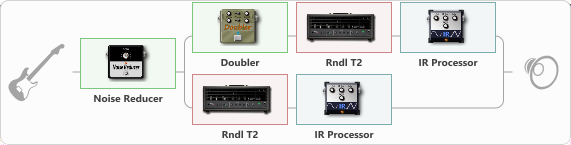# Pantera: Power Metal

Discussion in 'ToneLib-GFX presets' started by BGelais, Nov 29, 2022.

1. Pantera: Power Metal

Preset name: Pantera Power Metal

Stereo Rythm set with doubler. Enjoy!

Effects chain:Effect: "Noise Reducer" (Dynamics / Filter), active - "yes"
{
"Sens" = 80
"Mode" = Soft
}

Effect: "Splitter" (Dynamics / Filter)
{
"A-Bypass" = Off
"A-Pan" = -100
"A-Level" = 50
"B-Bypass" = Off
"B-Pan" = 100
"B-Level" = 50
"Width" = 100

'A' branch:
{

Effect: "Doubler" (Dynamics / Filter), active - "yes"
{
"A-Dly" = 75
"A-Inv" = Off
"A-Level" = 75
"B-Dly" = 50
"B-Inv" = Off
"B-Level" = 50
}

Effect: "Rndl T2" (Amp simulators), active - "yes"
{
"Gain" = 90
"Bass" = 50
"Middle" = 50
"Treble" = 50
"Presence" = 0
"Master" = 38
"Level (dB)" = 0
}

Effect: "IR Processor" (Cabinets), active - "yes"
{
"IR" = Pantera-Power_IR
"Low Cut (Hz)" = 0
"Hi Cut (kHz)" = 20.0
"Mix" = 100
"Level (dB)" = 0
}
}
'B' branch:
{

Effect: "Rndl T2" (Amp simulators), active - "yes"
{
"Gain" = 90
"Bass" = 50
"Middle" = 50
"Treble" = 50
"Presence" = 0
"Master" = 50
"Level (dB)" = -5
}

Effect: "IR Processor" (Cabinets), active - "yes"
{
"IR" = Pantera-Power_IR
"Low Cut (Hz)" = 0
"Hi Cut (kHz)" = 20.0
"Mix" = 100
"Level (dB)" = 0
}
}
}

Note: You will need to download and install the ToneLib-GFX software to use the preset.

#### Attached Files:

• ###### Pantera_Power_Metal.tlgfx
File size:
50.9 KB
Views:
2,865
fahad and Mezcal Sonics like this.